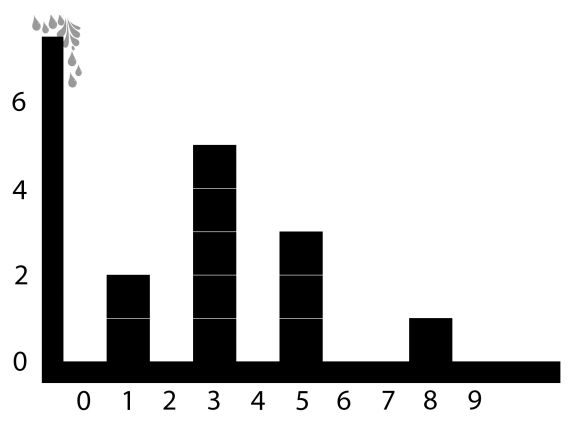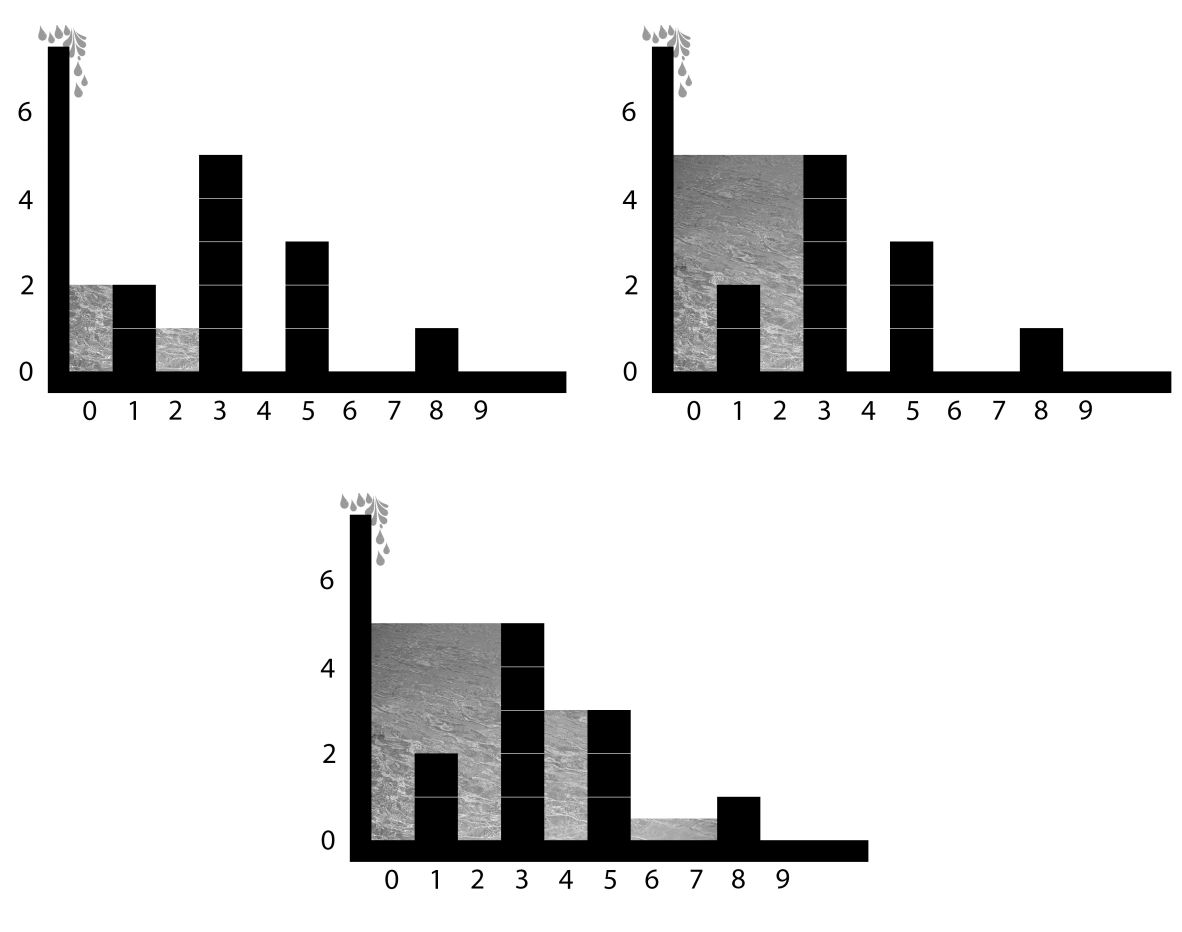시간 제한 메모리 제한 제출 정답 맞은 사람 정답 비율
2 초 512 MB 5 1 1 20.000%

문제

A big reservoir was built in Red river using a dam. Assume that the reservoir is a rectangular box with unit length width. The reservoir consists of many tanks. An example a cross section of an empty reservoir along its length and height dimensions is shown in the picture below:Water flows in from the top left gate into the reservoir. The tanks in the resevoir are constructed using water resistant walls. Each wall is one unit length thick (along the width dimention) and has its height smaller than the height of the reservoir.

Given the location and the height of the walls and the unit volume K of water flowing in, your task is to figure out the last wall water flows over.

입력

The input consists of several datasets. The first line of the input contains the number of datasets which is a positive integer and is not greater than 20. The following lines describe the datasets.

Each dataset is described by the following lines:

• The first line contains one positive integer N that is the number of walls separating the tanks (N ≤ 105).
• The second line contains N positive integers Li where Li is the horizonal location (along the length dimention of the reservoir) of the ith wall (1 ≤ Li ≤ 109, Li > Li-1 + 1 for i > 1).
• The third line contains N positive integers Hi where Hi is the height in unit length of the ith wall (1 ≤ Hi ≤ 105).
• The fourth line contains an integer Q that is a number of queries (Q ≤ 105).
• In the next Q lines, each line contains a positive integer K that is the unit volume of water flowing in the reservoir (K ≤ 1015)

출력

For each dataset, write out Q lines where the ith line contains the index of the last wall that water flows over for the ith query. If there is no wall that water flows over, output 0.

1
4
1 3 5 8
2 5 3 1
3
3
13
17

1
1
3

힌트

Explanations: